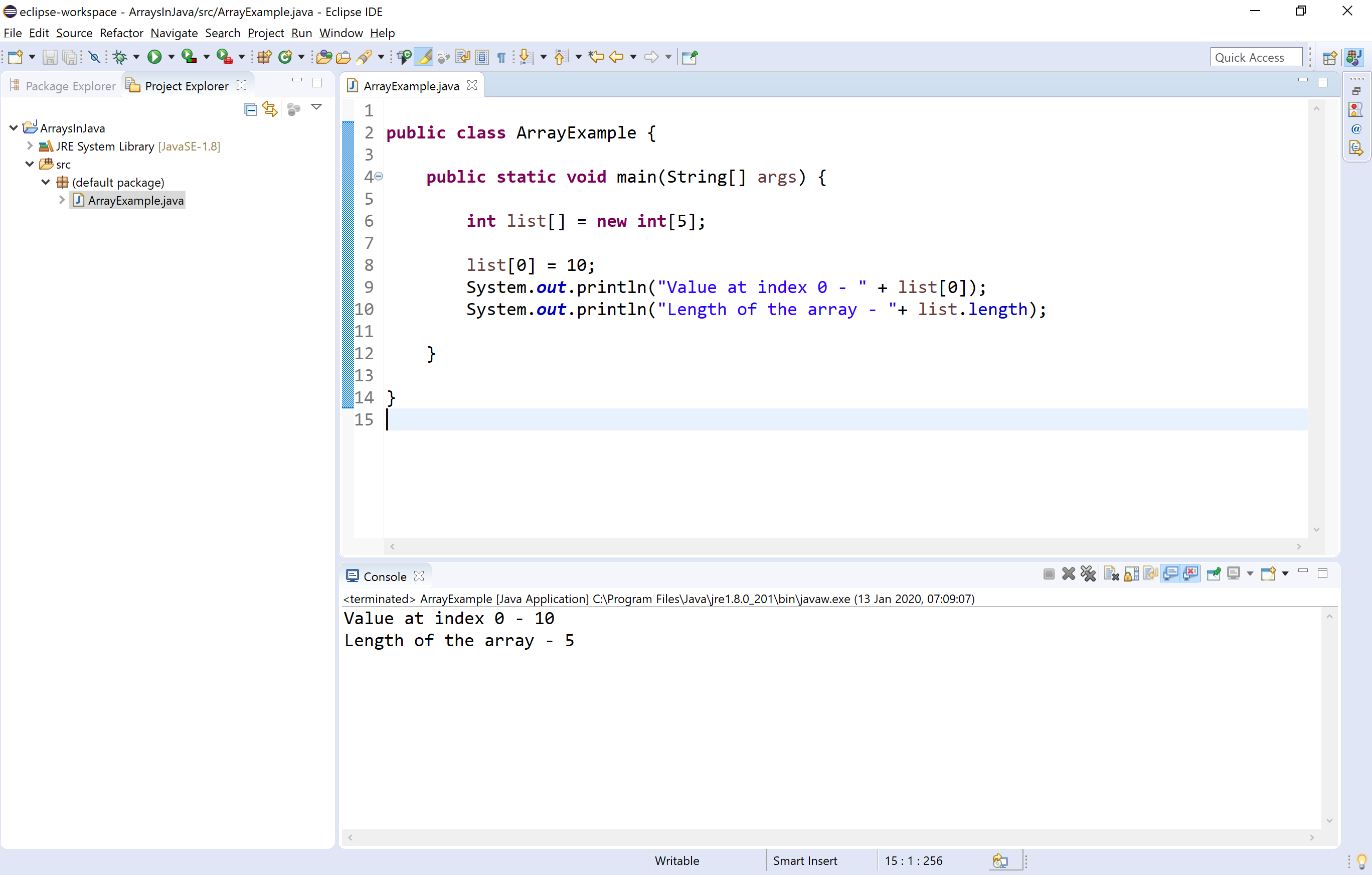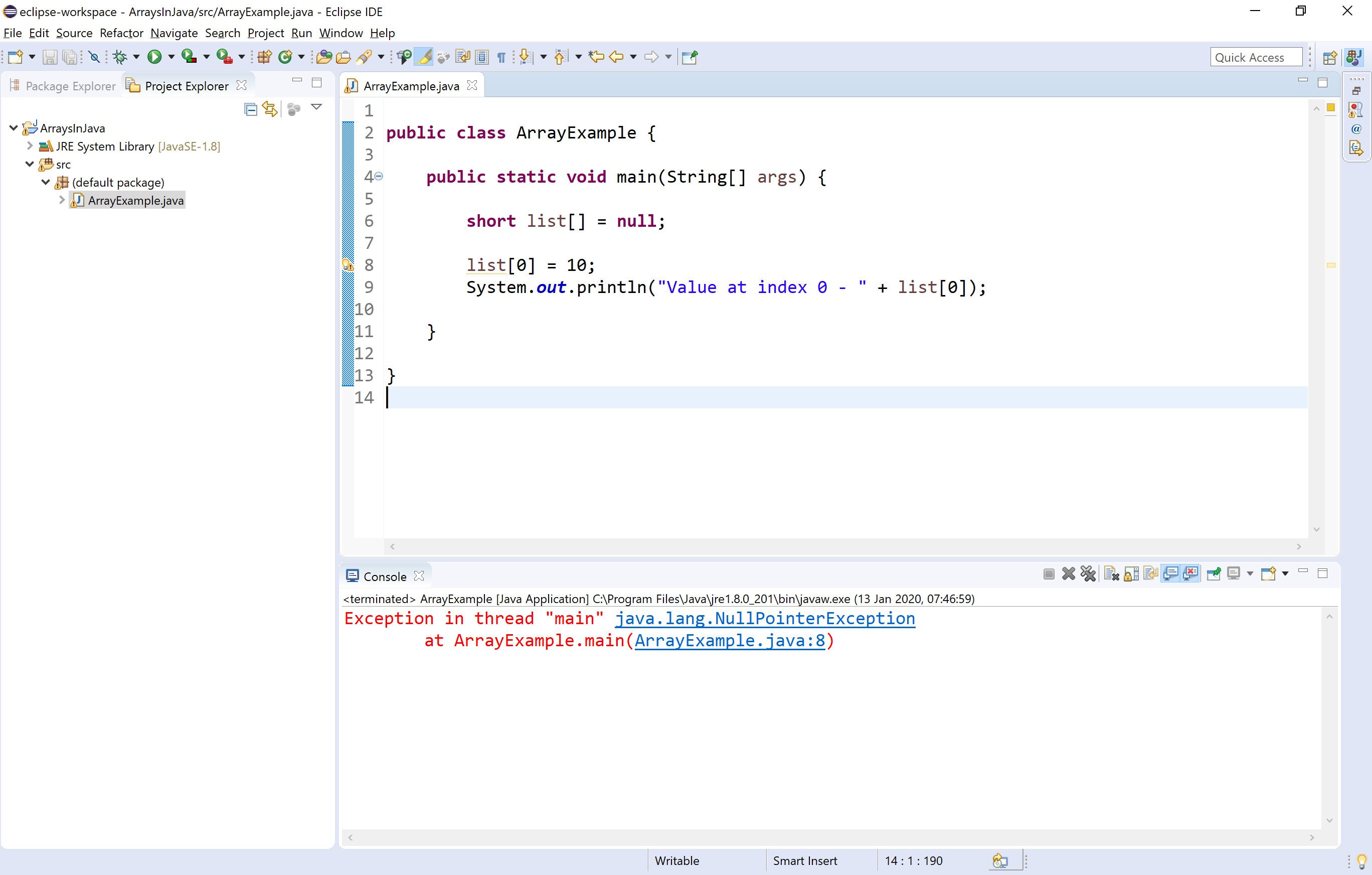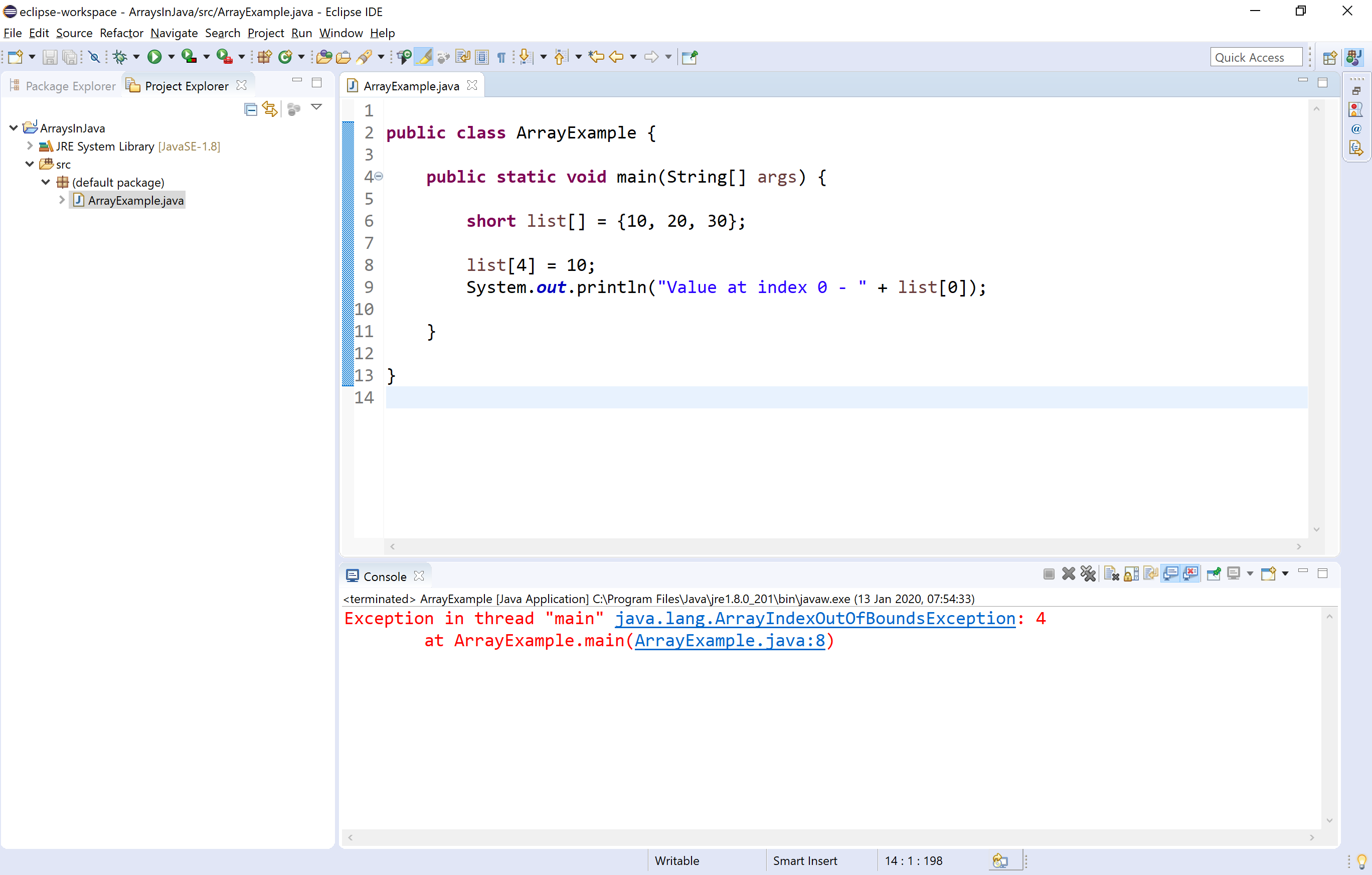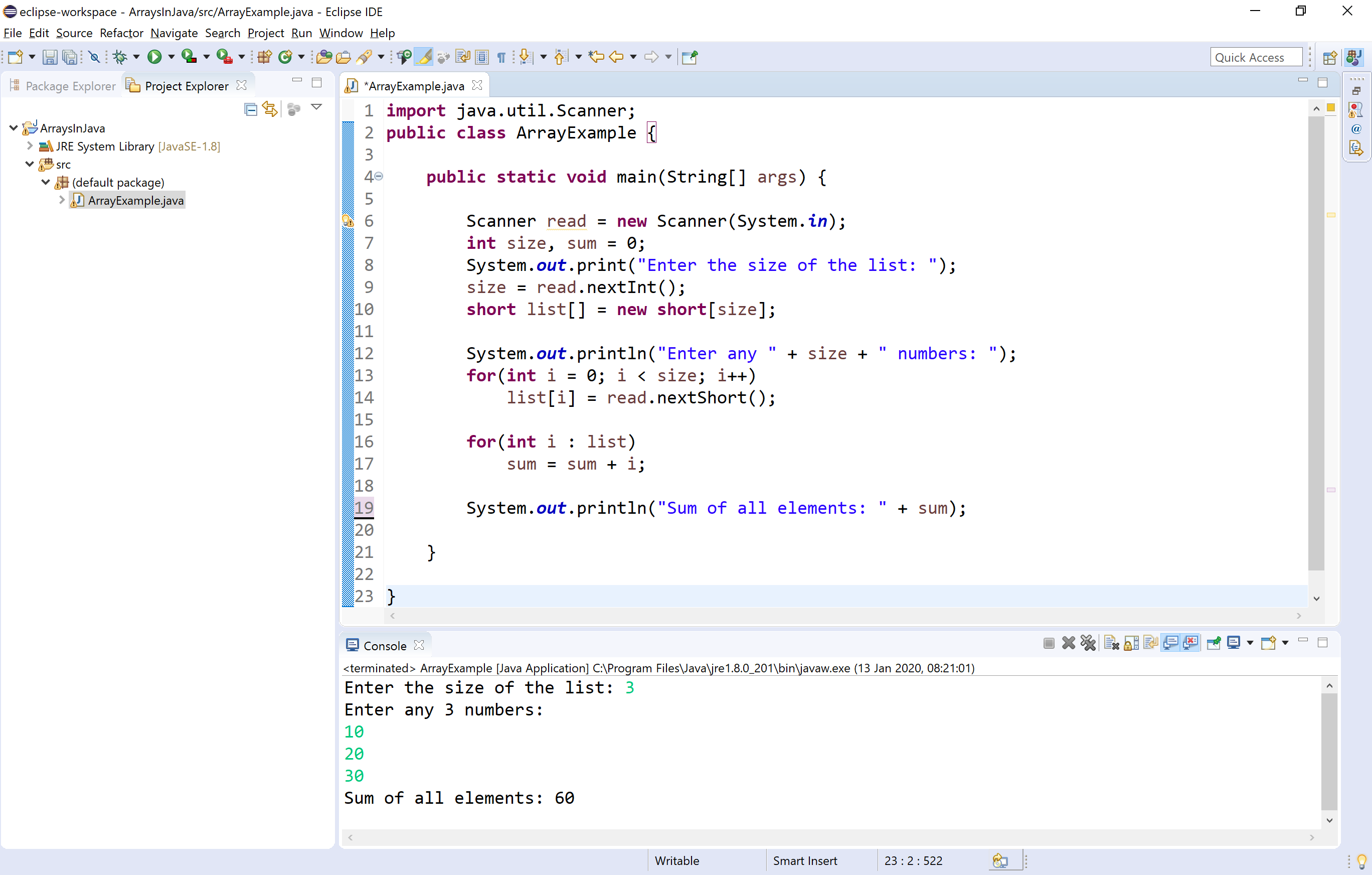The perfect place for easy learning...

×

# Java Arrays

An array is a collection of similar data values with a single name. An array can also be defined as, a special type of variable that holds multiple values of the same data type at a time.

In java, arrays are objects and they are created dynamically using new operator. Every array in java is organized using index values. The index value of an array starts with '0' and ends with 'zise-1'. We use the index value to access individual elements of an array.

In java, there are two types of arrays and they are as follows.

• One Dimensional Array
• Multi Dimensional Array

## Creating an array

In the java programming language, an array must be created using new operator and with a specific size. The size must be an integer value but not a byte, short, or long. We use the following syntax to create an array.

Syntax
``````data_type array_name[ ] = new data_type[size];
(or)
data_type[ ] array_name = new data_type[size];
``````

Let's look at the following example program.

Example
``````public class ArrayExample {

public static void main(String[] args) {

int list[] = new int;

list = 10;
System.out.println("Value at index 0 - " + list);
System.out.println("Length of the array - " + list.length);

}

}
``````

When we run the above example code, it produces the following output.In java, an array can also be initialized at the time of its declaration. When an array is initialized at the time of its declaration, it need not specify the size of the array and use of the new operator. Here, the size is automatically decided based on the number of values that are initialized.

Example
``````int list[ ] = {10, 20, 30, 40, 50};
``````

## NullPointerException with Arrays

In java, an array created without size and initialized to null remains null only. It does not allow us to assign a value. When we try to assign a value it generates a NullPointerException.

Look at the following example program.

Example
``````public class ArrayExample {

public static void main(String[] args) {

short list[] = null;

list = 10;
System.out.println("Value at index 0 - " + list);

}

}
``````

When we run the above example code, it produces the following output.## ArrayIndexOutOfBoundsException with Arrays

In java, the JVM (Java Virtual Machine) throws ArrayIndexOutOfBoundsException when an array is trying to access with an index value of negative value, value equal to array size, or value more than the array size.

Look at the following example program.

Example
``````public class ArrayExample {

public static void main(String[] args) {

short list[] = {10, 20, 30};

list = 10;
System.out.println("Value at index 0 - " + list);

}

}
``````

When we run the above example code, it produces the following output.## Looping through an array

An entire array is accessed using either simple for statement or for-each statement. Look at the following example program to display sum of all the lements in a list.

Example
``````import java.util.Scanner;
public class ArrayExample {

public static void main(String[] args) {

int size, sum = 0;
System.out.print("Enter the size of the list: ");
short list[] = new short[size];

System.out.println("Enter any " + size + " numbers: ");

for(int i = 0; i < size; i++)  // Simple for statement

for(int i : list)     // for-each statement
sum = sum + i;

System.out.println("Sum of all elements: " + sum);

}

}
``````

When we run the above example code, it produces the following output.## Multidimensional Array

In java, we can create an array with multiple dimensions. We can create 2-dimensional, 3-dimensional, or any dimensional array.

In Java, multidimensional arrays are arrays of arrays. To create a multidimensional array variable, specify each additional index using another set of square brackets. We use the following syntax to create two-dimensional array.

Syntax
``````data_type array_name[ ][ ] = new data_type[rows][columns];
(or)
data_type[ ][ ] array_name = new data_type[rows][columns];
``````

When we create a two-dimensional array, it created with a separate index for rows and columns. The individual element is accessed using the respective row index followed by the column index. A multidimensional array can be initialized while it has created using the following syntax.

Syntax
``````data_type array_name[ ][ ] = {{value1, value2}, {value3, value4}, {value5, value6},...};
``````

When an array is initialized at the time of declaration, it need not specify the size of the array and use of the new operator. Here, the size is automatically decided based on the number of values that are initialized.

Example
``````int matrix_a[ ][ ] = {{1, 2},{3, 4},{5, 6}};
``````

The above statement creates a two-dimensional array of three rows and two columns.